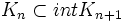# Hemicompact space

This article defines a property of topological spaces: a property that can be evaluated to true/false for any topological space|View a complete list of properties of topological spaces
This is a variation of compactness. View other variations of compactness

## Definition

### Symbol-free definition

A topological space is termed hemicompact if it is the union of an ascending sequence of compact subsets, each contained in the interior of the next, such that every compact subset is contained in one of these.

### Definition with symbols

A topological space$X$ is termed hemicompact if there is a sequence$K_n$ of compact subsets such that$K_n \subset int K_{n+1}$, and such that any compact subset is contained in$K_n$ for some$n$.